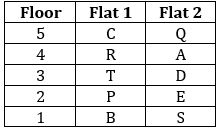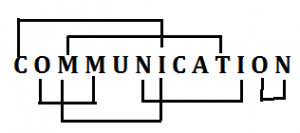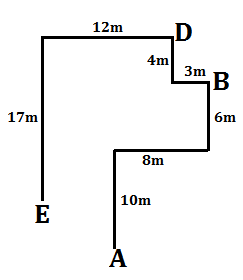Latest Banking jobs   »

# Reasoning Ability Quiz For SIDBI GRADE A 2023- 14th January

Direction (1-5): Study the following information carefully to answer the given questions:
Ten persons P, Q, R, S, T, A, B, C, D and E are living in a five storey building such that ground floor is numbered as 1, above it is floor 2 then top floor is numbered as 5. Each of the floor has 2 flats in it as flat-1 and flat-2. Flat-1 of floor-2 is immediately above flat-1 of floor-1 and immediately below flat-1 of floor-3 and so on. In the same way flat-2 of floor-2 is immediately above flat-2 of floor-1 and immediately below flat-2 of floor-3 and so on. Flat-1 is in west of flat-2.
Q lives in an odd numbered floor. Both T and B lives in the same flat number. R and A live in the same floor. C does not live in flat number 2. There is a gap of two floors in which Q and P lives. Both P and Q do not live in the same flat number. T lives immediately above P in the same flat number. There is a gap of one floor in which T and B lives. E who lives on an even numbered floor lives below A and both lives in the same flat number. D lives above S and both live in the same flat number. C and S neither live in the same flat number nor in the same floor.

Q1. Who among the following lives in flat-2 of 4th floor?
(a) R
(b) A
(c) P
(d) E
(e) None of these

Q2. Who among the following lives with Q in the same floor?
(a) C
(b) B
(c) E
(d) D
(e) None of these

Q3. How many floors are there between C and R?
(a) One
(b) Two
(c) None
(d) Three
(e) Cannot be determined

Q4. B lives on which of the following floor?
(a) Floor-1
(b) Floor-2
(c) Floor-3
(d) Floor-4
(e) Floor-5

Q5. Four of the following five are alike in a certain way and hence form a group, which among the following does not belong to that group?
(a) A
(b) E
(c) S
(d) D
(e) T

Q6. If all the letters in the word FUNDAMENTALS are arranged in alphabetical order from left to right in such a way that consonants are arranged first followed by vowels, then how many letters are there in between M and T after the arrangement?
(a) Two
(b) One
(c) None
(d) Three
(e) Four

Q7. How many pairs of letters are there in the word ‘COMMUNICATION’, each of which have as many letters between then in the word as they have between then in the English alphabet?
(a) One
(b) Four
(c) None
(d) Three
(e) More than Five

Q8. Which alphabet is 3rd from the left in the meaningful four-letter word formed from the first, fourth, seventh and tenth letter of the word ORGANIZATION? If more than one word is formed, then mark answer as X and no meaningful word is formed then mark answer as Z.
(a) T
(b) X
(c) P
(d) Z
(e) I

Q9. Which of the following elements should come in a place ‘?’ ?
AZ9 DW25 GT49 JQ81 ?
(a) NO141
(b) HS32
(c) MN121
(d) MN194
(e) None of these

Q10. If in the number 4567283691, 2 is added to first five digits of the number and 1 is subtracted from last five digits of the number, then how many digits are repeating in the number thus formed?
(a) Two
(b) One
(c) None
(d) Three
(e) Four

Q11. In a row of boys, Ashu is 18th from the right end and Yatin is 35th from the left end. If in this row Yatin is Fifteenth from the right then what is the position of Ashu from the left?
(a) 31
(b) 32
(c) 30
(d) 33
(e) None of these

Direction (12-13): Study the following information carefully and answer the given questions:
Five persons A, B, C, D and E travel in a car, each of them has different weight. More than two persons are lighter than D. B is heavier than A but lighter than E. No one lighter than C. D is not the heaviest person.

Q12. Who among the following is heaviest person?
(a) C
(b) D
(c) E
(d) A
(e) B

Q13. If weight of third lightest person is 58kg, what is possible weight of A?
(a) 60kg
(b) 75kg
(c) 62kg
(d) 55kg
(e) 70kg

Directions (14-15): Study the information and answer the given questions:
A person starts walking from point A towards north and walk 10m, then turn right and walks 8m, from there he walks 6m north and reach point B. From point B he walks 3m left and 4m right respectively and reach point D. From point D he turns left and walk 12m and then take a left turn and walks 17m to reach final point E.

Q14. What is the shortest distance in between initial point and final point?
(a) 14m
(b) 10m
(c) 4m
(d) 5m
(e) None of these

Q15. What is the direction of point D with respect of point A?
(a) North-East
(b) South
(c) South-West
(d) North
(e) West

Solutions

Solutions (1-5):
Sol.S1. Ans. (b)
S2. Ans. (a)
S3. Ans. (c)
S4. Ans. (a)
S5. Ans. (e)

S6. Ans. (d)
Sol. Original Word: FUNDAMENTAL
Obtained Word: DFLMNNSTAAEU

S7. Ans. (e)
Sol.S8. Ans. (d)

S9. Ans. (c)

S10. Ans. (a)
Sol. Original Number: 4567283691
Obtained Number: 6789472580

S11. Ans. (b)
Solution: Clearly, Ashu is 18th from the right end and
Yatin is 35th from the left end and 15th from the right end of the row
So, number of boys in the row = (35 -1 + 15) = 49
Now, Ashu is 18th from the right
Ashu from left end= 49- (18-1) = 32
Hence, Ashu is 32th from the left end of the row.

Solutions (12-13):
Sol. E > D > B > A > C

S12. Ans. (c)
S13. Ans. (d)

Solution (14-15):
Sol.S14. Ans. (e)
S15. Ans. (a)## FAQs

### When will the SIDBI Grade A Exam 2023 be held?

The SIDBI Grade A Exam 2023 will be held on 28th January 2023.

#### Congratulations!